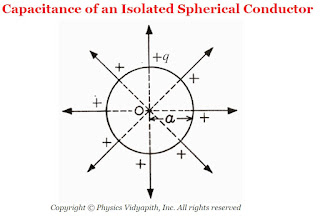### Capacitance of an Isolated Spherical Conductor

Derivation →Capacitance of an Isolated Spherical Conductor

Let us consider an isolated spherical conductor of radius $a$ is placed in a vacuum or air. Let a charge $+q$ be given to the sphere and this charge is distributed uniformly on the surface of conducting sphere. Then the electric potential at the surface of the conducting sphere is

$V=\frac{1}{4\pi \epsilon_{\circ}} \frac{q}{a} \qquad(1)$

So the capacitance of the sphere

$C=\frac{q}{V} \qquad(2)$

Now substitute the value of $V$ in equation $(2)$ Then we get

$C=\frac{q}{\frac{q}{4\pi \epsilon_{\circ}a}}$

 $C=4\pi \epsilon_{\circ}a$

Thus, The capacitance of a spherical conductor is directly proportional to its radius. i.e If the radius of conducting sphere is large then the sphere will hold a large amount of the given charge without running up too high a voltage.

Unit: The unit of capacitance is "farad" i.e. $F$